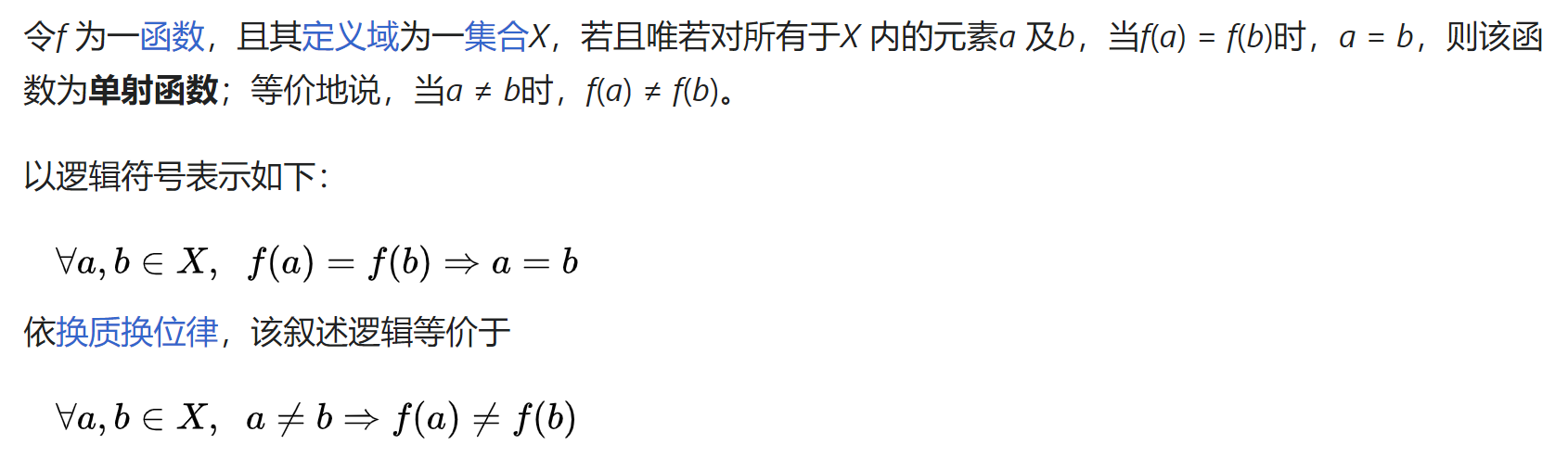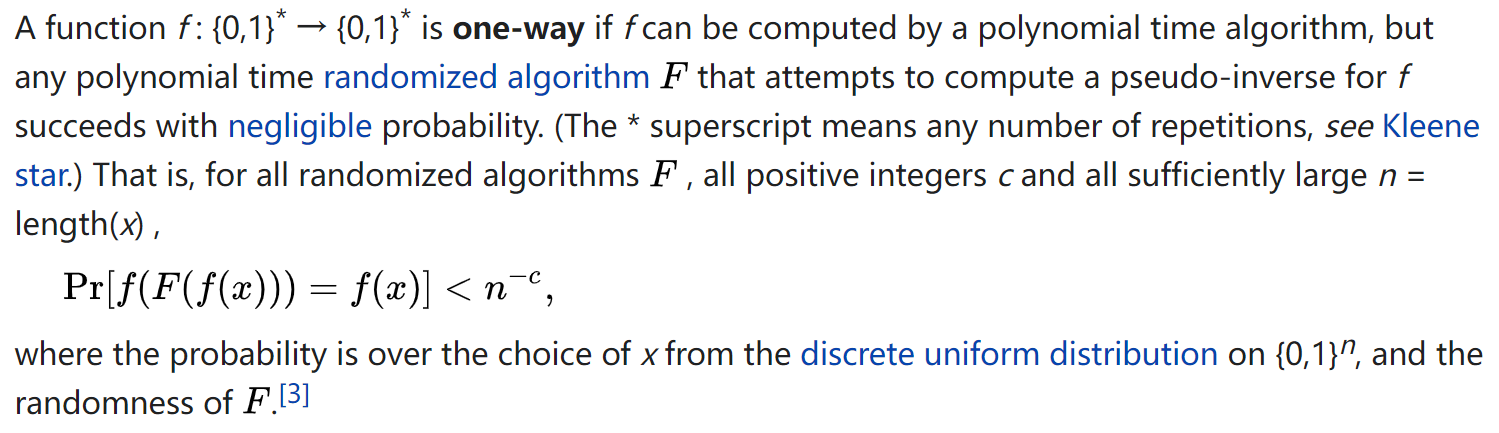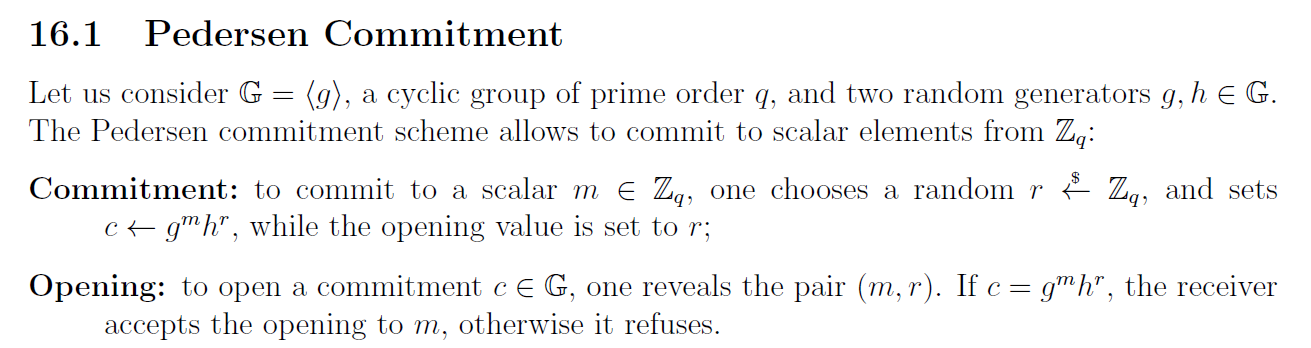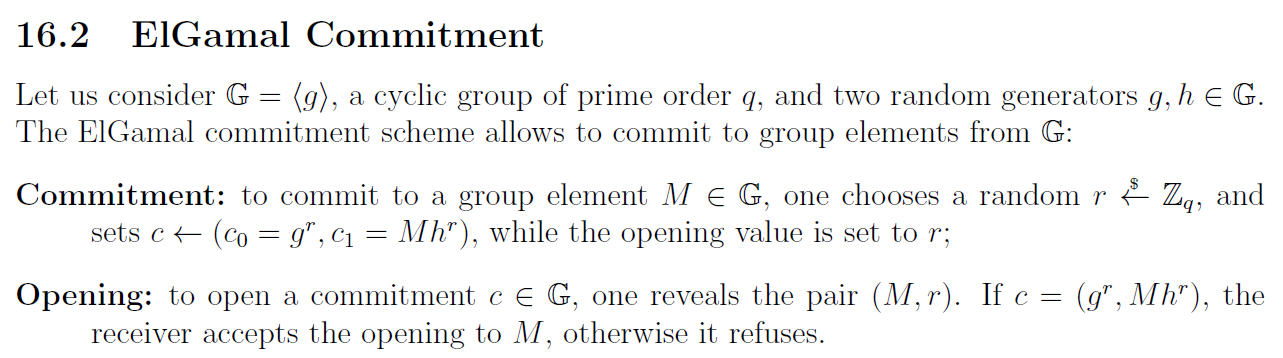# 定义与安全定义·

## 简介——承诺和打开·

$\begin{array}{|c|} \hline \\ \begin{array}{ccc} \mathrm{Committer}(m) & \ \ \ \ \ \ \ \ \ \ \ \ \ \ & \mathrm{Receiver} \\ \begin{array}{l} (c, d) \leftarrow \rm{Commit}(m, r) \end{array} & & \\ & \overset{c}{\longrightarrow} & \\ \vdots & & \\ & \overset{m,\ d}{\longrightarrow} & \\ & & \begin{array}{l} \rm{Open(c, m, d)} \rightarrow \text{Accept/Reject} \end{array} \\ \end{array} \\ \hline \end{array}$

## 安全定义——绑定和隐藏·

• Binding：不存在$m' \ne m$，使得$\rm{Commit}(m, r)=\rm{Commit}(m', r')$
• Hiding：令$\mathcal{R}$是所有随机数的集合，对于所有$m' \ne m$，都有$\{\rm{Commit}(m, \mathcal{R})\} \equiv \{\rm{Commit}(m', \mathcal{R})\}$

Perfect的安全性显然比Computational的高，但坏消息是，Binding和Hiding是不能同时做到Perfect的，这个可以简单从定义上推出，即不存在$m' \ne m$，使得$\rm{Commit}(m, r)=\rm{Commit}(m', r')$的话，就不会有$\{\rm{Commit}(m, \mathcal{R})\} \equiv \{\rm{Commit}(m', \mathcal{R})\}$；相似地，若对于所有$m' \ne m$，都有$\{\rm{Commit}(m, \mathcal{R})\} \equiv \{\rm{Commit}(m', \mathcal{R})\}$，即会存在$m' \ne m$，使得$\rm{Commit}(m, r)=\rm{Commit}(m', r')$。也可参考这里

## 后门——提取和模棱两可·

• 提取（extractability）即在知道某个后门的情况下可以从$c$提取出$m$
• 模棱两可（equivocality）即在知道某个后门的情况下根据$m, r, m' \ne m$可以计算出$r'$使得$c$$m'$的承诺，让接收方Open时由$c=\rm{Commit}(m, r)$接受$m'$

• 密钥生成：$\rm{KeyGen}(1^k) \rightarrow (pk, td)$，这里的$1^k$是安全参数；
• 提取：$(c, d) \leftarrow \rm{Commit_{pk}(m, r)}$，则$\rm{Extra_{td}(c)} \rightarrow m$
• 模棱两可：$(c, d) \leftarrow \rm{Commit_{pk}(m, r)}$，给定$m' \ne m$，则$\rm{Equiv_{td}(m, r, m')} \rightarrow (r', d')$，使得$(c, d') \leftarrow \rm{Commit}_{pk}(m', r')$$\rm{Open}(c, m', d')=\rm{Accept}$。（PS：其实很多文章都是说输出$r'$的，不过套我这上一节的定义的话这里是$d'$，感觉输出$d'$更加广义，输出$r'$$d'=r'$时的一种特殊情况）。

# 从函数性质的角度看·

## 单射->Perfectly Binding？·• $\forall r, \forall m, m' \in \mathcal{M}, m \ne m' \Rightarrow \rm{Commit}_r(m) \ne \rm{Commit}_r(m')$
• $\forall r, r’ \in \mathcal{R}, r \ne r’ \Rightarrow {\rm{Commit_r(\mathcal{M})}} \cap {\rm{Commit_{r’}(\mathcal{M})}} = \empty$。

（PS：当然，如果看成是$\rm{Commit}_m$的函数簇也是可以的，反正最后给不同的$(m,r)$可以射到不同的点就好了。另挖坑有空补个图）

$\begin{array}{|c|} \hline \\ \begin{array}{ccc} \mathrm{Committer}(m) & \ \ \ \ \ \ \ \ \ \ \ \ \ \ & \mathrm{Receiver} \\ \begin{array}{l} r \overset{\}{\leftarrow} \mathcal{R} \\ c = F_r(m) \\ b = \rm{Bind}(r) \end{array} & & \\ & \overset{b,\ c}{\longrightarrow} & \\ \vdots & & \\ & \overset{m,\ r}{\longrightarrow} & \\ & & \begin{array}{l} b \overset{?}{=} \rm{Bind}(r) \\ c \overset{?}{=} F_r(m) \end{array} \\ \end{array} \\ \hline \end{array}$

## 满射->Perfect Hiding？·

• $\forall m \in \mathcal{M}, \{\rm{Commit_m(\mathcal{R})}\} = \mathcal{C}$

• $\forall m \in \mathcal{M}, \forall c \in \mathcal{C}, \exists r \in \mathcal{R}, s.t. \rm{Commit}_m(r)=c$

$\begin{array}{|c|} \hline \\ \begin{array}{ccc} \mathrm{Committer}(m) & \ \ \ \ \ \ \ \ \ \ \ \ \ \ & \mathrm{Receiver} \\ \begin{array}{l} r \overset{\}{\leftarrow} \mathcal{R} \\ c = F_m(r) \\ \end{array} & & \\ & \overset{c}{\longrightarrow} & \\ \vdots & & \\ & \overset{m,\ r}{\longrightarrow} & \\ & & \begin{array}{l} c \overset{?}{=} F_m(r) \end{array} \\ \end{array} \\ \hline \end{array}$

Hiding的证明在上面分析的基础上其实是显然的，但是这时Binding就没这么显然了（或者说其实这样构造的话根本没有Binding？）

## OWF->Computational？·

OWF即上面提到过的One-way Function，简单来说是一类正向求解容易，逆向求解困难的函数，准确点说则是：在多项式时间内求出伪逆（Pseudo-inverse）的概率可以忽略（换句话说就是计算上不可能），以下摘抄了wiki上的定义。## 双射TF->Extractability/Equivocality？·

• $(pk, td) \leftarrow \rm{TFGen}(1^k)$
• $y = \rm{TFInvert}_{td}(\rm{TF}_{pk}(x))$，其中$\rm{TF}_{pk}(x)=\rm{TF}_{pk}(y)$

# 栗子·

## 我瞎编的栗子·

### Perfect Hinding 的栗子·

$\begin{array}{|c|} \hline \\ \begin{array}{ccc} \mathrm{Committer}(m) & \ \ \ \ \ \ \ \ \ \ \ \ \ \ & \mathrm{Receiver} \\ \begin{array}{l} r \overset{\}{\leftarrow} \mathcal{R} \\ c = \rm{Per}_m( \rm{TF}_{pk}(r) ) \\ \end{array} & & \\ & \overset{c}{\longrightarrow} & \\ \vdots & & \\ & \overset{m,\ r}{\longrightarrow} & \\ & & \begin{array}{l} c \overset{?}{=} \rm{Per}_m( \rm{TF}_{pk}(r) ) \end{array} \\ \end{array} \\ \hline \end{array}$

• Perfect Hiding：简单转换一下可得$\rm{Commit}(m, \mathcal{R})=\rm{Per}_m( \rm{TF}_{pk}(\mathcal{R}) )$，由于$\rm{TF}_{pk}$双射，即$\rm{Commit}(m, \mathcal{R})=\rm{Per}_m(\mathcal{T} )$，由于排列肯定双射，即$\rm{Commit}(m, \mathcal{R})=\mathcal{C}$，所以对于所有$m' \ne m$，都有$\{\rm{Commit}(m, \mathcal{R})\} \equiv \{\rm{Commit}(m', \mathcal{R})\} = \mathcal{C}$
• Computational Binding：假设给定$(m, r)$和足够随机（反正$m'$攻击者不可控，应该也合理？）的$m' \ne m$，攻击者可以找到$r'$使得$\rm{Commit}(m', r')=\rm{Commit(m, r)}$，即使得$\rm{Per}_{m'}( \rm{TF}_{pk}(r') ) = \rm{Per}_m( \rm{TF}_{pk}(r) )$，即使得$r' = \rm{TF}_{pk}^{-1}( \rm{Per}_{m'}^{-1}( \rm{Per}_m( \rm{TF}_{pk}(r) )))$，由于$m'$足够随机，即$\rm{Per}_{m'}^{-1}( \rm{Per}_m( \rm{TF}_{pk}(r) ))$足够随机，攻击者在不知道trapdoor的情况下对一个Trapdoor Function求一个足够随机的数的逆，计算上不可能；
• Equivocality：与Binding的证明类似，但模拟者可以知道$td$，也即可以通过$r' = \rm{TFInvert}_{td}^{-1}( \rm{PerInvert}_{m'}( \rm{Per}_m( \rm{TF}_{pk}(r) )) )$求得$r'$实现模棱两可。由于$\rm{TF}$是双射的，所以总能求得这样的$r'$

（PS：不好实例化。。。）

### Perfect Binding的栗子·

$\begin{array}{|c|} \hline \\ \begin{array}{ccc} \mathrm{Committer}(m) & \ \ \ \ \ \ \ \ \ \ \ \ \ \ & \mathrm{Receiver} \\ \begin{array}{l} r \overset{\}{\leftarrow} \mathcal{R} \\ c = m \bigoplus r \\ b = \rm{Bind}_{pk}( r ) \end{array} & & \\ & \overset{b,\ c}{\longrightarrow} & \\ \vdots & & \\ & \overset{m,\ r}{\longrightarrow} & \\ & & \begin{array}{l} b \overset{?}{=} \rm{Bind}_{pk}( r ) \\ c \overset{?}{=} m \bigoplus r \end{array} \\ \end{array} \\ \hline \end{array}$

• Perfect Binding：由于$\rm{Bind}_{pk}$单射，$\rm{Bind}_{pk}(r) = \rm{Bind}_{pk}(r') \Rightarrow r = r'$，所以不存在$r' \ne r$，使得$b \overset{?}{=} \rm{Bind}_{pk}( r )$通过；假设存在$m' \ne m$使得$c = m \bigoplus r = m' \bigoplus r$，则$m = m' = c \bigoplus r$，矛盾，所以不存在$m' \ne m$使得$c \overset{?}{=} m \bigoplus r$通过；综上，不存在$m' \ne m$使得Open时输出Accept。
• Computational Hiding：假设攻击者可以根据$b$$c$算出$m$，则可以算出$r = c \bigoplus m$从而在没有$td$的情况下根据$b = \rm{Bind}_{pk}( r )$算出$r$，规约到TF上计算不可能；
• Extractability：给定$b$$c$，模拟这可以使用$td$计算出$r = \rm{BindInvert}_{td}(b)$，然后$m = c \bigoplus r$

## 比较著名的栗子·

Perfect Hiding的Pedersen Commitment：Perfect Binding的ElGamal Commitment：# 参考·

[CF01] Canetti R, Fischlin M. Universally composable commitments[C]//Annual International Cryptology Conference. Springer, Berlin, Heidelberg, 2001: 19-40.

[DN02] Damgård I, Nielsen J B. Perfect hiding and perfect binding universally composable commitment schemes with constant expansion factor[C]//Annual International Cryptology Conference. Springer, Berlin, Heidelberg, 2002: 581-596.

… …